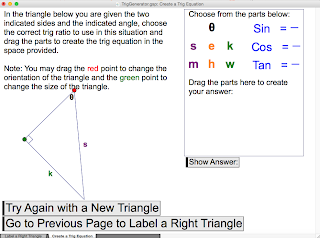## Sunday, 28 February 2016

### Geometer's Sketchpad - Trig Ratio GeneratorWhen using the Geometer's Sketchpad (for both computer and iPad) it is often better to "start from sketch, not from scratch". That is, give students a premade sketch rather having them build something from nothing (as many textbooks would have you do).
In this activity, students can practice two very specific skills dealing with trigonometry. The first is simply being able to correctly place the names of the sides of a right triangle (opposite, adjacent and hypotenuse). Students drag the side names and then can check their answers and then randomly generate another triangle to try again. The second is one where a random triangle is generated that shows information about two sides and one angle. Students then drag parts of an equation to create a trig ratio equation. They can check their answer and then randomly generate other right angled triangle to try again.
This is not meant to be something that a student uses for a long length of time but instead just some quick practice to re enforce the basic ideas from trig ratios.